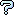All about flooble | fun stuff | Get a free chatterbox | Free JavaScript | Avatarsperplexus dot infoThree tasks (Posted on 2014-08-21)Given a 12-member set of consecutive integers (1,2,3…11,12).
Partition it into 3 subsets, each with a distinct number of members ,
e.g . A=(1,2); B=(3,5,11,12) & C=(4,6,7,8,9,10).
Evaluate the sums of the members for each of the subsets, in our example:
Sa=3 ; Sb=31 & Sc=44;

Now evaluate:
F1 (product of the three sums) = Sa * Sb * Sc, i.e. 3*31*44 = 4092 in our example.

F2= Sa * Sb + Sc; equals to 93+44=137 in our case.

F3= d(Sa) +d(Sb) + d(Sc); where d(N) is the number of N's divisors.
So in our case F3=2+2+6 = 10.

The 3 independent tasks :

Executing the above procedures find the partitions of the main set, that provide maximum values for F1, F2 & F3.

 No Solution Yet Submitted by Ady TZIDON No RatingComments: ( You must be logged in to post comments.)
 Subject Author Datecomputer solutions Charlie 2014-08-21 16:53:45re: Are null sets allowed? Ady TZIDON 2014-08-21 15:54:12Are null sets allowed? Steve Herman 2014-08-21 15:01:58Please log in:

 Search: Search body:
Forums (1)